## 旋转中心刚体-FGM梁刚柔热耦合动力学特性研究 1)

* 江苏科技大学机械工程学院, 江苏镇江212003

** 扬州大学建筑科学与工程学院, 江苏扬州 225127

## RESEARCH ON DYNAMICS OF A RIGID-FLEXIBLE-THERMAL COUPLING ROTATING HUB-FGM BEAM 1)

Fan Jihua,*,2), Chen Liwei*, Wang Mingqiang*, Zhang Dingguo, Du Chaofan**

* School of Mechanical Engineering, Jiangsu University of Science and Technology, Zhenjiang 212003, Jiangsu, China

School of Sciences, Nanjing University of Science and Technology, Nanjing 210094, China

** College of Civil Science and Engineering, Yangzhou University, Yangzhou 225127, Jiangsu, China

 基金资助: 1) 国家自然科学基金.  115020981) 国家自然科学基金.  117721581) 国家自然科学基金.  11802263 江苏省自然科学基金.  BK20180895江苏省高校自然科学研究面上项目.  15KJB130003江苏科技大学博士科研启动基金资助项目.  120140003

Received: 2019-08-28   Accepted: 2019-10-22   Online: 2019-10-22Abstract

The rigid-flexible-thermal coupling dynamics of the hub-FGM beam system under large overall rotating motion is studied. The FGM beam is an Euler-Bernoulli beam, and its physical properties follow certain kinds of power law gradient distribution and vary in the thickness direction. The longitudinal deformation and the transverse deformation of the flexible beam are considered and the coupling term of the deformation which is caused by transverse deformation of the flexible beam is included in the expression of longitudinal deformation. Considering the influence of the thermal coupling of the tapered hollow beam which is under the condition of external high temperature and internal cooling passage cooling on the dynamic characteristics of the system, the temperature field distributed along the thickness direction of the FGM beam is obtained, and the thermal strain is included in the constitutive relationship of the beam. By using the assumed mode method to describe the deformation of the beam, the rigid-flexible-thermal coupling dynamic equations of the system are derived via employing Lagrange's equations of the second kind, as well as to compile the dynamics simulation software. Then the dynamics of the system are studied through simulation examples. The results show that the dynamic response of beams with different sections is quite different, so it is necessary to model the actual system reasonably. When the large overall rotating motion is known, the FGM beam considering the thermal shock load will effectively suppress the transverse bending deformation, and the high-frequency oscillation will occur with the superposition of the thermal shock when the large overall rotating motion is constant; When the large overall rotating motion is unknown, the external torque and the thermal shock load interact to produce a thermal coupling effect, which causes the system to exhibit high-frequency oscillation, at the same time , the rigid-flexible thermal coupling effect of the hub-FGM beam system is appeared.

Keywords： functionally graded material ; tapered hollow beam ; rigid-flexible-thermal coupling ; dynamic simulation

Fan Jihua, Chen Liwei, Wang Mingqiang, Zhang Dingguo, Du Chaofan. RESEARCH ON DYNAMICS OF A RIGID-FLEXIBLE-THERMAL COUPLING ROTATING HUB-FGM BEAM 1). Chinese Journal of Theoretical and Applied Mechanics[J], 2019, 51(6): 1905-1917 DOI:10.6052/0459-1879-19-241

## 1 旋转中心刚体柔性梁动力学模型

### 图1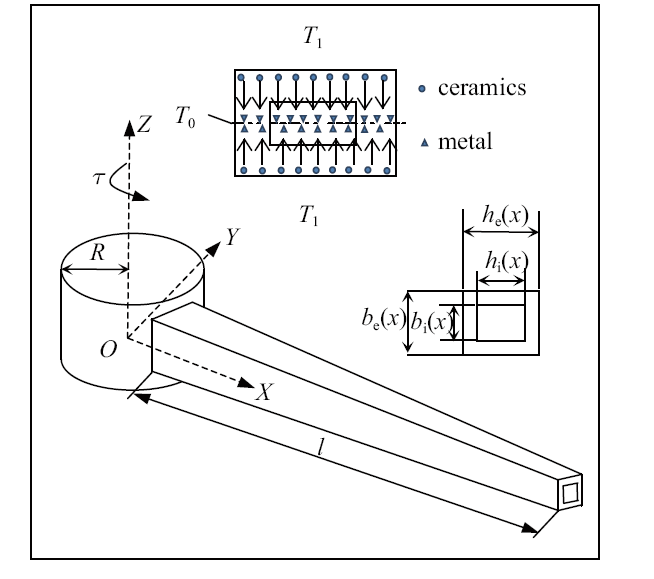Fig.1   Physical model of the hub-FGM beam with internal cooling passage

FGM梁材料分布是两侧耐高温的陶瓷(ceramics)材料按幂律变化至中间金属(metal)材料的形式.

$\begin{eqnarray}Q(y) = (Q_{\rm c} - Q_{\rm m} )\left| {\frac{2y}{h}} \right|^N + Q_{\rm m}\end{eqnarray}$

$\begin{eqnarray}&&h_{\rm e} (x) = h_{\rm e} - \frac{x}{l}(h_{\rm e} - {h}'_{\rm e} ) =h_{\rm e} \left( {1 - \frac{x}{l}g_{\rm 1} } \right)\end{eqnarray}$
$\begin{eqnarray}\\&&b_{\rm e} (x) = b_{\rm e} - \frac{x}{l}(b_{\rm e} - {b}'_{\rm e} ) =b_{\rm e} \left( {1 - \frac{x}{l}g_{\rm 2} } \right)\end{eqnarray}$
$\begin{eqnarray}\\&&h_{\rm i} (x) = h_{\rm i} - \frac{x}{l}(h_{\rm i} - h_{\rm i} ^\prime)=h_{\rm i} \left( {1 - \frac{x}{l}g_{\rm 3} } \right)\end{eqnarray}$
$\begin{eqnarray}\\&&b_{\rm i} (x) = b_{\rm i} - \frac{x}{l}(b_{\rm i} - b_{\rm i} ^\prime ) =b_{\rm i} \left( {1 - \frac{x}{l}g_{\rm 4} } \right)\end{eqnarray}$

$$\begin{eqnarray*}&&g_{\rm 1} =1 - \frac{{h}'_{\rm e} }{h_{\rm e} },\ \ g_{\rm 2} =1 -\frac{{b}'_{\rm e} }{b_{\rm e} }\\&&g_{\rm 3} =1 - \frac{h_{\rm i} ^\prime }{h_{\rm i}},\ \ g_{\rm 4} = 1 - \frac{b_{\rm i} ^\prime}{b_{\rm i} }\end{eqnarray*}$$

### 1.2 热传导方程

$\begin{eqnarray}\frac{\rm d}{{\rm d}y}\left[ {K\left( y \right)\frac{{\rm d}T(y)}{{\rm d}y}} \right] = 0\end{eqnarray}$

$\begin{eqnarray}\left. \begin{array}{ll} T = T_1,\ \ & y = \pm \dfrac{h_{\rm e} (x)}{2} \\ T = T_0, &y = 0 \\\end{array} \right\} \end{eqnarray}$

$\begin{eqnarray}T_{\rm a} (y) = T_0 + \frac{\Delta T}{c_1}\int_0^{|y|}{1}\Bigg/\lt(K_{{\rm cm}} \left| {\frac{2y}{h_{\rm e} }} \right|^N + K_{\rm m}) {\rm d}y \end{eqnarray}$

$\begin{eqnarray}&&T_{\rm a} (y) = T_0 + \frac{\Delta T}{c_1 }\Bigg[\left| {\frac{2y}{h_{\rm e} }} \right| - \frac{K_{{\rm cm}} }{(N + 1)K_{\rm m} }\left| {\frac{2y}{h_{\rm e} }} \right|^{N + 1} +\\&&\qquad \frac{K_{{\rm cm}}^2 }{(2N + 1)K_{\rm m}^2 }\left| {\frac{2y}{h_{\rm e} }} \right|^{2N + 1} - \frac{K_{{\rm cm}}^3 }{(3N + 1)K_{\rm m}^3 }\left| {\frac{2y}{h_{\rm e} }} \right|^{3N + 1} +\\&&\qquad \frac{K_{{\rm cm}}^4 }{(4N + 1)K_{\rm m}^4 }\left| {\frac{2y}{h_{\rm e} }} \right|^{4N + 1} - \frac{K_{{\rm cm}}^5 }{(5N + 1)K_{\rm m}^5 }\left| {\frac{2y}{h_{\rm e} }} \right|^{5N + 1}\Bigg] \end{eqnarray}$

$\begin{eqnarray}&&c_1 = \Bigg[1 - \frac{K_{{\rm cm}} }{(N + 1)K_{\rm m} } + \frac{K_{{\rm cm}}^2 }{(2N + 1)K_{\rm m}^2 } - \frac{K_{{\rm cm}}^3}{(3N + 1)K_{\rm m}^3 } +\\&&\qquad \frac{K_{{\rm cm}}^4 }{(4N + 1)K_{\rm m}^4 } - \frac{K_{{\rm cm}}^5}{(5N + 1)K_{\rm m}^5 }\Bigg] \end{eqnarray}$

$\begin{eqnarray}\left. {{\begin{array}{*{20}c} {T = T_1 ,y = \pm \dfrac{h_{\rm e} (x)}{2}} \\\\{T = T_0 ,y = \pm \dfrac{h_{\rm i} (x)}{2}}\\\end{array} }} \right\}\end{eqnarray}$

$\begin{eqnarray}T_{\rm b} (y) = T_0 + \frac{\Delta T}{c_2 }\int_{\left| {\frac{h_{\rm i} }{2}} \right|}^{\left| y \right|} {1}\Bigg/\Bigg({K_{{\rm cm}} \left| {\frac{2y}{h_{\rm e} }} \right|^N + K_{\rm m}}\Bigg){\rm d}y \end{eqnarray}$

$\begin{eqnarray} &&T_{\rm b} (y) = T_0 + \frac{T}{c_2 }\Bigg\{\left| {\frac{2y}{h_e }} \right| - \left( {\frac{h_{\rm i} }{h_e }} \right) -\\&&\qquad \frac{K_{{\rm cm}} }{(N + 1)K_{\rm m} }\Bigg[\left| {\frac{2y}{h_{\rm e} }} \right|^{N + 1} - \left( {\frac{h_{\rm i} }{h_e }} \right)^{N + 1}\Bigg] +\\&&\qquad \frac{K_{{\rm cm}}^2 }{(2N + 1)K_{\rm m}^2 }\Bigg[\left| {\frac{2y}{h_{\rm e} }} \right|^{2N + 1} - \left( {\frac{h_{\rm i} }{h_{\rm e} }} \right)^{2N + 1}\Bigg] -\\&&\qquad \frac{K_{{\rm cm}}^3 }{(3N + 1)K_{\rm m}^3 }\Bigg[\left| {\frac{2y}{h_{\rm e} }} \right|^{3N + 1} - \left( {\frac{h_{\rm i} }{h_{\rm e} }} \right)^{3N + 1}\Bigg] +\\&&\qquad \frac{K_{{\rm cm}}^4 }{(4N + 1)K_{\rm m}^4 }\Bigg[\left| {\frac{2y}{h_{\rm e} }} \right|^{4N + 1} - \left( {\frac{h_{\rm i} }{h_{\rm e} }} \right)^{4N + 1}\Bigg] -\\&&\qquad \frac{K_{{\rm cm}}^5 }{(5N + 1)K_{\rm m}^5 }\Bigg[\left| {\frac{2y}{h_{\rm e} }} \right|^{5N + 1} - \left( {\frac{h_{\rm i} }{h_{\rm e} }} \right)^{5N + 1}\Bigg]\Bigg\} \end{eqnarray}$

$\begin{eqnarray} \label{eq14} && c_2 = 1 - \left( {\frac{h_{\rm i} }{h_{\rm e} }} \right) - \frac{K_{{\rm cm}} }{(N + 1)K_{\rm m} }\Bigg[1 - \left( {\frac{h_{\rm i} }{h_{\rm e} }} \right)^{N + 1}\Bigg] +\\&&\qquad \frac{K_{{\rm cm}}^2 }{(2N + 1)K_{_{\rm m} }^{\rm 2} }\Bigg[1 - \left( {\frac{h_{\rm i} }{h_{\rm e} }} \right)^{2N + 1}\Bigg] -\\&&\qquad \frac{K_{{\rm cm}}^3 }{(3N + 1)K_{_{\rm m} }^{\rm 3} }\Bigg[1 - \left( {\frac{h_{\rm i} }{h_{\rm e} }} \right)^{3N + 1}\Bigg] +\\&&\qquad \frac{K_{{\rm cm}}^4 }{(4N + 1)K_{_{\rm m} }^{\rm 4} }\Bigg[1 - \left( {\frac{h_{\rm i} }{h_{\rm e} }} \right)^{4N + 1}\Bigg] -\\&&\qquad \frac{K_{{\rm cm}}^5 }{(5N + 1)K_{_{\rm m} }^{\rm 5} }\Bigg[1 - \left( {\frac{h_{\rm i} }{h_{\rm e} }} \right)^{5N + 1}\Bigg] \end{eqnarray}$

### 图2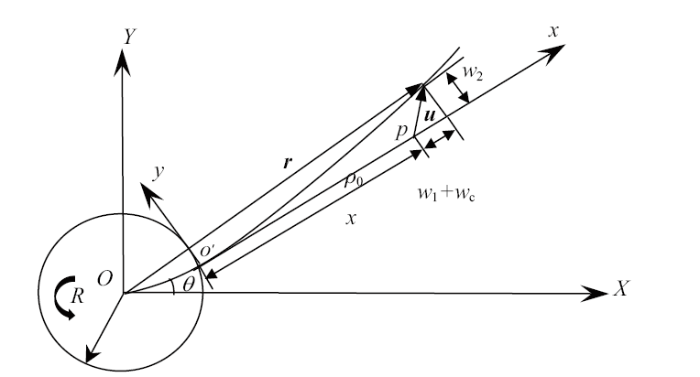Fig.2   The schematic diagram of flexible beam deformation

$\begin{eqnarray} r = r_{\rm 0} + \rho _0 + u \end{eqnarray}$

$\begin{eqnarray} \label{eq16} \left. {{\begin{array}{l} {u_x \left( {x,t} \right) = w_1 + w_{\rm c} } \\ {u_y \left( {x,t} \right) = w_2 } \\ {w_{\rm c} \left( {x,t} \right) = - \dfrac{1}{2}\displaystyle\int_0^x {\left( {\dfrac{\partial w_2 }{\partial \zeta }} \right)^2{\rm d}\zeta } } \\ \end{array} }} \right\} \end{eqnarray}$

$\begin{eqnarray} \label{eq17} && r = \varTheta \left( { r_0 + \rho _0 + u} \right) =\\&&\qquad \left( {{\begin{array}{*{20}c} {\cos \theta } & { - \sin \theta } \\ {\sin \theta } & {\cos \theta } \\ \end{array} }} \right)\left( {{\begin{array}{*{20}c} {R + x + w_1 + w_{\rm c} } \\ {w_2 } \\ \end{array} }} \right) \end{eqnarray}$

$\begin{eqnarray} T_k =\frac{1}{2}J_{\rm oh} \dot{\theta }^2 + \frac{1}{2}\int_V {\rho \left( y \right)} \dot{ r}^{\rm T}\dot{ r}{\rm d}V \end{eqnarray}$

$\begin{eqnarray}U=\frac12\int_V {E(y)\varepsilon _x^2 {\rm d}V}\end{eqnarray}$

$\varepsilon _x$为梁上任意点的轴向应变

$\begin{eqnarray}\varepsilon _x = \frac{\partial w_1 }{\partial x} - y\frac{\partial ^2w_2}{\partial x^2}\end{eqnarray}$

$\begin{eqnarray} \sigma = E(y)(\varepsilon _x - \alpha (y)\Delta T) \end{eqnarray}$

$\begin{eqnarray} \left. {{\begin{array}{*{20}c} {w_1 (x,t) = \varPhi _x (x) A(t)} \\ {w_2 (x,t) = \varPhi _y (x) B(t)} \\ \end{array} }} \right\} \end{eqnarray}$

$\begin{eqnarray} w_{\rm c} = - \frac{1}{2} B^{\rm T} H(x) B \end{eqnarray}$

$\begin{eqnarray} && \delta W_{\rm T} = \int_V {\delta \varepsilon _x \sigma _{\rm T} } =\\&&\qquad \int_V {\delta \varepsilon _x } E(y)( - \alpha (y)\Delta T){\rm d}V = \delta Q_{\rm T}^{\rm T} \delta q \end{eqnarray}$

$\begin{eqnarray} && Q_{{\rm Ta}} = \Bigg[0,\int_0^{\left| {\frac{h_{\rm e} }{2}} \right|} {E(y)b_{\rm e} (x)\alpha (y)} \Delta T{\rm d}y\int_0^l {{\varphi }'_x {\rm d}x} , - \\&&\qquad \int_0^{\left| {\frac{h_{\rm e} }{2}} \right|} {yE(y)b_{\rm e} (x)\alpha (y)} \Delta T{\rm d}y\int_0^l {{\varphi }''_y {\rm d}x} \Bigg]^{\rm T}\end{eqnarray}$
$\begin{eqnarray} && Q_{{\rm Tb}} = \Bigg[0,\int_{\left| {\frac{h_{\rm i} }{2}} \right|}^{\left| {\frac{h_{\rm e} }{2}} \right|} {E(y)b_{\rm i} (x)\alpha (y)} \Delta T{\rm d}y\int_0^l {{\varphi }'_x {\rm d}x} , -\\&&\qquad \int_{\left| {\frac{h_{\rm i} }{2}} \right|}^{\left| {\frac{h_{\rm e} }{2}} \right|} {yE(y)b_{\rm i} (x)\alpha (y)}\Delta T{\rm d}y\int_0^l {{\varphi }''_y {\rm d}x} \Bigg]^{\rm T} \end{eqnarray}$

$\begin{eqnarray} \frac{\rm d}{{\rm d}t}\left( {\frac{\partial T_k }{\partial \dot{q}}} \right) - \frac{\partial T_k }{\partial q} = - \frac{\partial U}{\partial q} + Q_{\rm T} + F_q \end{eqnarray}$

$\begin{eqnarray} \left( {{\begin{array}{*{20}c} {M_{11} } & { M_{12} } & { M_{13} } \\ { M_{21} } & { M_{22} } & { M_{23} } \\ { M_{31} } & { M_{32} } & { M_{33} } \\ \end{array} }} \right)\left( {{\begin{array}{*{20}c} \ddot{\theta } \\ \ddot{ A} \\ \ddot{ B} \\ \end{array} }} \right) = \left[ {{\begin{array}{*{20}c} {Q_{\rm \theta } } \\ { Q_{\rm A} } \\ { Q_{\rm B} } \\ \end{array} }} \right] \end{eqnarray}$

$\begin{eqnarray}&& M_{11} = J_{{\rm oh}} + J_{{\rm ob}} + 2 S_x A + A^{\rm T} M_x A + \\&&\qquad B^{\rm T} M_y B - B^{\rm T} C B + \\&&\qquad \frac{1}{4}\Bigg(\int_0^l {\rho b_{\rm e} (x)h_{\rm e} (x)(\underline{ B^{\rm T} H B B^{\rm T} H B})} {\rm d}x- \\&&\qquad \int_0^l {\rho b_{\rm i} (x)h_{\rm i} (x)(\underline{ B^{\rm T} H B B^{\rm T} H B})} {\rm d}x\Bigg) -\\&&\qquad \Bigg(\int_0^l {\rho b_{\rm e} (x)h_{\rm e} (x)(\underline{ A^{\rm T} \varPhi_x^{\rm T} B^{\rm T} H B})} {\rm d}x -\\&&\qquad \int_0^l {\rho b_{\rm i} (x)h_{\rm i} (x)(\underline{ A^{\rm T} \varPhi _x^{\rm T} B^{\rm T} H B})} {\rm d}x\Bigg)\\\end{eqnarray}$
$\begin{eqnarray} && M_{22}= M_{x}\end{eqnarray}$
$\begin{eqnarray} && M_{33} = M_y + \int_0^l {\rho b_{\rm e} (x)h_{\rm e} (x)(\underline{ H B B^{\rm T} H})} {\rm d}x -\\&&\qquad \int_0^l {\rho b_{\rm i} (x)h_{\rm i} (x)(\underline{ H B B^{\rm T} H})} {\rm d}x \\ \end{eqnarray}$
$\begin{eqnarray}&& M_{21} = M_{12}^{\rm T} = - M_{xy} B \end{eqnarray}$
$\begin{eqnarray} && M_{31} = M_{13}^{\rm T} = S_y^{\rm T} + M_{{xy} }^{\rm T} A + \\&&\qquad \int_0^l {\rho b_{\rm e} (x)h_{\rm e} (x)\underline{ H B \varPhi _y B^{\rm T}}} {\rm d}x -\\&&\qquad \frac{1}{2}\int_0^l {\rho b_{\rm e} (x)h_{\rm e} (x)\underline{ H \varPhi _{_y }^{\rm T} B^{\rm T} H B}} {\rm d}x -\\&&\qquad \Bigg(\int_0^l {\rho b_{\rm i} (x)h_{\rm i} (x)\underline{ H B \varPhi _y B^{\rm T}}} {\rm d}x -\\&&\qquad \frac{1}{2}\int_0^l {\rho b_{\rm i} (x)h_{\rm i} (x)\underline{ H \varPhi _{_y }^{\rm T} B^{\rm T} H B}} {\rm d}x\Bigg) \\ \end{eqnarray}$
$\begin{eqnarray} && M_{32} = M_{{23}}^{\rm T} = - \Bigg(\int_0^l {\rho b_{\rm e} (x)h_{\rm e} (x)\underline{ H B \varPhi _x }} {\rm d}x-\\&&\qquad \int_0^l {\rho b_{\rm i} (x)h_{\rm i} (x)\underline{ H B \varPhi _x }} {\rm d}x\Bigg)\\ \end{eqnarray}$
$\begin{eqnarray}&& Q_ \theta=\tau-2\dot{\theta}[ S_x\dot{ A}+ A^{\rm T} M_x\dot{ A}+ B^{\rm T} M_y\dot{ B}- B^{\rm T} \underline{ C}\dot{ B}] -\\&&\qquad \Bigg[\int_0^l \rho b_{\rm e} (x)h_{\rm e} (x) \underline{ B^{\rm T} \varPhi _y^{\rm T} \dot{ B}^{\rm T} H\dot{ B}}{\rm d}x - \\&&\qquad \int_0^l \rho b_{\rm i} (x)h_{\rm i} (x) \underline{ B^{\rm T} \varPhi_y^{\rm T} \dot{ B}^{\rm T} H\dot{ B}}{\rm d}x\Bigg] +\\&&\qquad \dot{\theta }\Bigg[\int_0^l \rho b_{\rm e} (x)h_{\rm e} (x) \underline{\dot{ A}^{\rm T} \varPhi _x^{\rm T} B^{\rm T} H B}{\rm d}x -\\&&\qquad \int_0^l \rho b_{\rm i} (x)h_{\rm i} (x) \underline{\dot{ A}^{\rm T} \varPhi _x^{\rm T} B^{\rm T} H B}{\rm d}x\Bigg] + \\&&\qquad \dot{\theta }\int_0^l \rho b_{\rm e} (x)h_{\rm e} (x) (\underline{2 A^{\rm T} \varPhi _x^{\rm T} B^{\rm T} H\dot{ B}}){\rm d}x -\\&&\qquad \dot{\theta}\int_0^l \rho b_{\rm e} (x)h_{\rm e} (x) (\underline{ B^{\rm T} H B B^{\rm T} H\dot{ B}}){\rm d}x -\\&&\qquad \dot{\theta }\Bigg[\int_0^l \rho b_{\rm i} (x)h_{\rm i} (x) (\underline{2 A^{\rm T} \varPhi _x^{\rm T} B^{\rm T} H\dot{ B}}){\rm d}x -\\&&\qquad \int_0^l \rho b_{\rm i} (x)h_{\rm i} (x) (\underline{ B^{\rm T} H B B^{\rm T} H\dot{ B}}){\rm d}x\Bigg]\\ \end{eqnarray}$
$\begin{eqnarray} && Q_A = \dot{\theta }^2( S_x^{\rm T} + M_x A) + 2\dot{\theta } M_{xy} \dot{ B} - K_1 A + K_2 B - \\&&\qquad\frac{1}{2}\dot{\theta }^2\Bigg[\int_0^l {\rho b_{\rm e} (x)h_{\rm e} (x)(\underline{ \varPhi _x^{\rm T} B^{\rm T} H B})} {\rm d}x -\\&&\qquad \int_0^l {\rho b_{\rm i} (x)h_{\rm i} (x)(\underline{ \varPhi _x^{\rm T} B^{\rm T} H B})} {\rm d}x\Bigg] +\\&&\qquad \int_0^l {\rho b_{\rm e} (x)h_{\rm e} (x)(\underline{ \varPhi _x^{\rm T} \dot{ B}^{\rm T} H\dot{ B}})} {\rm d}x -\\&&\qquad \int_0^l {\rho b_{\rm i} (x)h_{\rm i} (x)(\underline{ \varPhi _x^{\rm T} \dot{ B}^{\rm T} H\dot{ B}})} {\rm d}x - K_4 \\ \end{eqnarray}$
$\begin{eqnarray}&& Q_B = \dot{\theta }^2(\underline{ M_y - C}) B - 2\dot{\theta } M_{xy} \dot{ A} - K_3 B + K_2^{\rm T} A + \\&&\qquad\dot{\theta }^2\int_0^l {\rho b_{\rm e} (x)h_{\rm e} (x)\Bigg(\underline{\frac{1}{2} H B B^{\rm T} H B - H B\varPhi _x A}\Bigg)} {\rm d}x -\\&&\qquad \dot{\theta }^2\int_0^l {\rho b_{\rm i} (x)h_{\rm i} (x)\Bigg(\underline{\frac{1}{2} H B B^{\rm T} H B - H B \varPhi _x A}\Bigg)} {\rm d}x -\\&&\qquad 2\dot{\theta }\Bigg[\int_0^l {\rho b_{\rm e} (x)h_{\rm e} (x)(\underline{ H B \varPhi _y \dot{ B} - \varPhi _y^{\rm T} B^{\rm T} H\dot{ B}})} {\rm d}x -\\&&\qquad \int_0^l {\rho b_{\rm i} (x)h_{\rm i} (x)(\underline{ H B \varPhi _y \dot{ B} - \varPhi _y^{\rm T} B^{\rm T} H\dot{ B}})} {\rm d}x\Bigg] -\\&&\qquad \int_0^l \rho \lt[b_{\rm e} (x)h_{\rm e} (x) - b_{\rm i} (x)h_{\rm i} (x)]\cdot\\&&\qquad(\underline{ H B\dot{ B}^{\rm T} H\dot{ B}}) {\rm d}x + K_5 \end{eqnarray}$

$\begin{eqnarray}&&\rho = 2\int_{\frac{h_{\rm i} }{2}}^{\frac{h_{\rm e} }{2}} {\rho (y)}{\rm d}y\end{eqnarray}$
$\begin{eqnarray} \\&&J_{\rm ob}=\int_0^l\rho[b_{\rm e}(x)h_{\rm e}(x)-b_{\rm i}(x)h_{\rm i}(x)](R+x)^2{\rm d}x\end{eqnarray}$
$\begin{eqnarray}\\&& S_x = \int_0^l \rho [b_{\rm e} (x)h_{\rm e} (x) - b_{\rm i} (x)h_{\rm i} (x)]\cdot\\&&\qquad(R + x)\varPhi _x (x) {\rm d}x \end{eqnarray}$
$\begin{eqnarray}\\&& S_y = \int_0^l \rho [b_{\rm e} (x)h_{\rm e} (x) - b_{\rm i} (x)h_{\rm i} (x)]\cdot\\&&\qquad(R + x)\varPhi _y (x) {\rm d}x \end{eqnarray}$
$\begin{eqnarray}\\&& M_x = \int_0^l \rho [b_{\rm e} (x)h_{\rm e} (x) - b_{\rm i} (x)h_{\rm i} (x)]\cdot\\&&\qquad\varPhi _x^{\rm T} (x)\varPhi _x (x){\rm d}x\end{eqnarray}$
$\begin{eqnarray}\\&& M_y = \int_0^l {\rho b_{\rm e} (x)h_{\rm e} (x)\varPhi _y^{\rm T} (x)\varPhi _y } (x){\rm d}x - \\&&\qquad \int_0^l {\rho b_{\rm i} (x)h_{\rm i} (x)\varPhi _y^{\rm T} (x)\varPhi _y } (x){\rm d}x \end{eqnarray}$
$\begin{eqnarray}\\&& M_{xy} = \int_0^l \rho [b_{\rm e} (x)h_{\rm e} (x) - b_{\rm i} (x)h_{\rm i} (x)]\cdot\\&&\qquad\varPhi _x^{\rm T} (x)\varPhi _y (x){\rm d}x\end{eqnarray}$
$\begin{eqnarray}\\&& C = \int_0^l \rho [b_{\rm e} (x)h_{\rm e} (x) - b_{\rm i} (x)h_{\rm i} (x)]\cdot\\&&\qquad(R + x) H(x){\rm d}x\end{eqnarray}$
$\begin{eqnarray}\\&& K_{\rm 1} = \int_0^l {E_{\rm 1} {\varPhi'}_x^{\rm T} (x)} {\varPhi'}_x {\rm d}x\end{eqnarray}$
$\begin{eqnarray}\\&& K_{\rm 2} = \int_0^l {E_2 {\varPhi'}_x (x)} {{\varPhi''}}_y (x){\rm d}x\end{eqnarray}$
$\begin{eqnarray}\\&& K_{\rm 3} = \int_0^l {E_3 {\varPhi''}_y^{\rm T} (x){\varPhi''}_y (x)} {\rm d}x\end{eqnarray}$
$\begin{eqnarray}\\&& K_4 = \int_0^l {E_4 } {\varPhi'}_x {\rm d}x\end{eqnarray}$
$\begin{eqnarray}\\&& K_5 = \int_0^l {E_5 } {\varPhi''}_y {\rm d}x\end{eqnarray}$
$\begin{eqnarray}\\&& E_{\rm 1} = \int_0^{\left| {\frac{h_{\rm e} }{2}} \right|} {E(y)(} b_{\rm e} - b_{\rm i} ){\rm d}x + \int_{\left| {\frac{h_{\rm i} }{2}} \right|}^{\left| {\frac{h_{\rm e} }{2}} \right|} {E(y)b_{\rm i} } {\rm d}x\end{eqnarray}$
$\begin{eqnarray}\\&&E_{\rm 2} = \int_0^{\left| {\frac{h_{\rm e} }{2}} \right|} {E(y)y(} b_{\rm e} - b_{\rm i} ){\rm d}x + \int_{\left| {\frac{h_{\rm i} }{2}} \right|}^{\left| {\frac{h_{\rm e} }{2}} \right|} {E(y)yb_{\rm i} } {\rm d}x\end{eqnarray}$
$\begin{eqnarray}\\&&E_{\rm 3} = \int_0^{\left| {\frac{h_{\rm e} }{2}} \right|} {E(y)y^2(} b_{\rm e} - b_{\rm i} ){\rm d}x + \int_{\left| {\frac{h_{\rm i} }{2}} \right|}^{\left| {\frac{h_{\rm e} }{2}} \right|} {E(y)y^2b_{\rm i} } {\rm d}x\end{eqnarray}$
$\begin{eqnarray}\\&& E_4 = \underline{\underline {\int_{\left| {\frac{h_i }{2}} \right|}^{\left| {\frac{h_{\rm e} }{2}} \right|} {E(y){b}_{\rm e} (x)\alpha } (y)(T_{\rm a} (y) - T_0 ){\rm d}y}} + \\&&\qquad\underline{\underline{\underline {\int_0^{\left| {\frac{h_{\rm e} }{2}} \right|} {E(y)[b_{\rm e} (x) - b_{\rm i} (x)]\alpha (y)} (T_{\rm b} (y) - T_0 ){\rm d}y}} }\end{eqnarray}$
$\begin{eqnarray}\\&& E_5 = \underline{\underline {\int_{\left| {\frac{h_{\rm i} }{2}} \right|}^{\left| {\frac{h_{\rm e} }{2}} \right|} {E(y){b}_{\rm e} (x)\alpha } (y)[T_{\rm a} (y) - T_0 ]y{\rm d}y}} +\\&&\qquad \underline{\underline{\underline {\int_0^{\left| {\frac{h_{\rm e} }{2}} \right|} {E(y)[b_{\rm e} (x) - b_{\rm i} (x)]\alpha (y)} (T_b (y) - T_0 )y{\rm d}y}} }\\&& \end{eqnarray}$

## 2 动力学仿真

### 2.1 大范围运动已知的动力学仿真

$\begin{eqnarray}\dot{\theta} = \left\{\begin{array}{l@{\quad} l} \dfrac{\omega _0 }{T_{\rm t} }t - \dfrac{\omega _0 }{2{\pi}}\sin \left( \dfrac{2\pi}{T_{\rm t}}t \right), & 0 \leqslant t \leqslant T_{\rm t} \\ \omega _0, & t > T_{\rm t}\\ \end{array}\right. \end{eqnarray}$

### 图3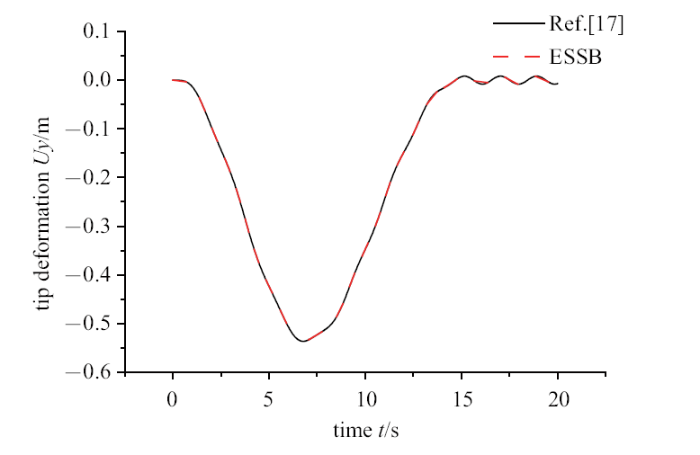Fig.3   Contrast verification

### 图4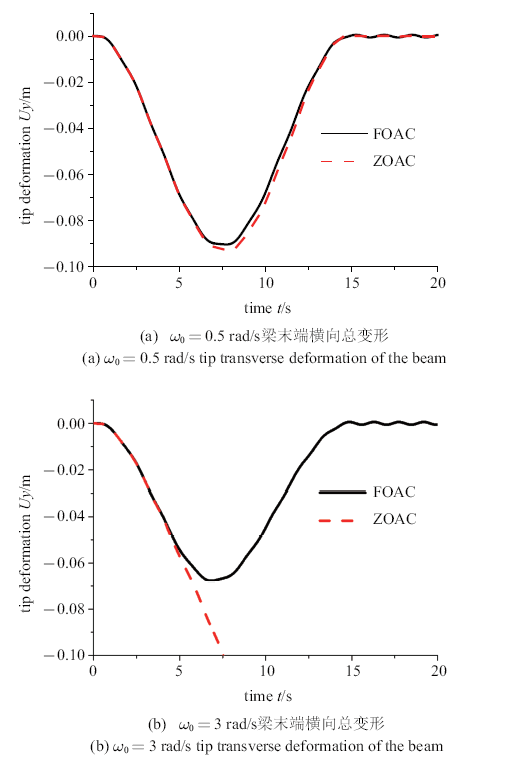Fig.4   Comparison of a FOAC model and a ZOAC model

### 图5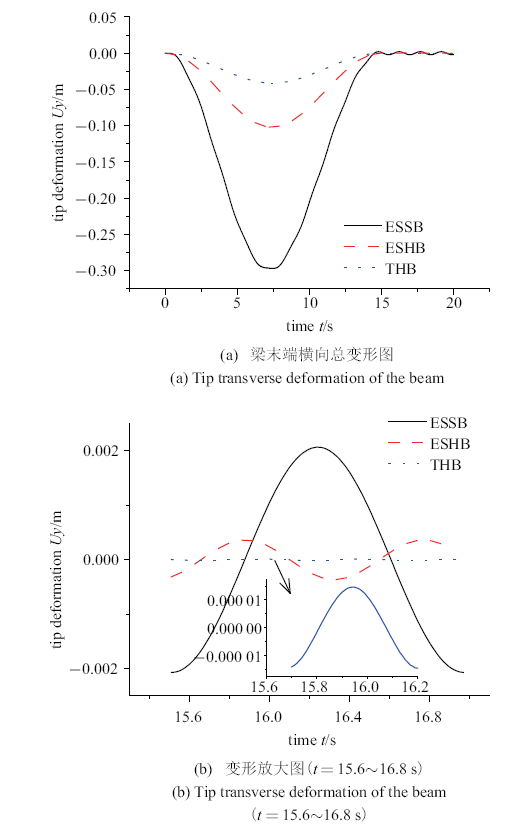Fig.5   Comparison of tip deformation of flexible beam in three different models

### 图6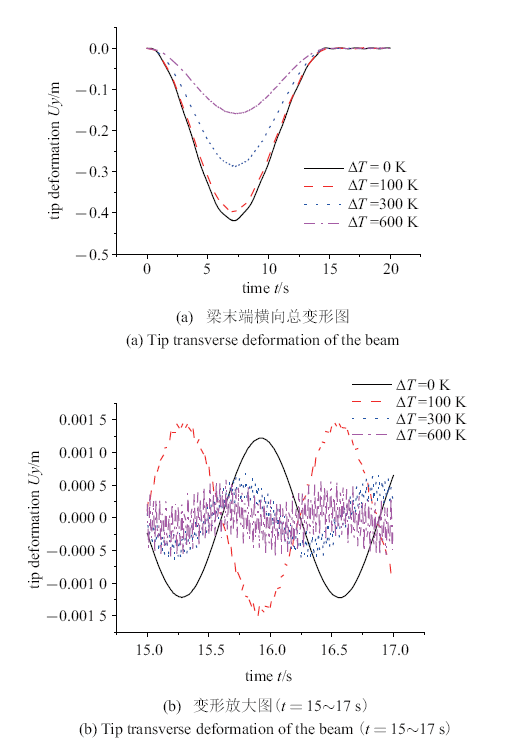Fig.6   Tip deformation of flexible beam under the influence of the different thermal shock load

### 图7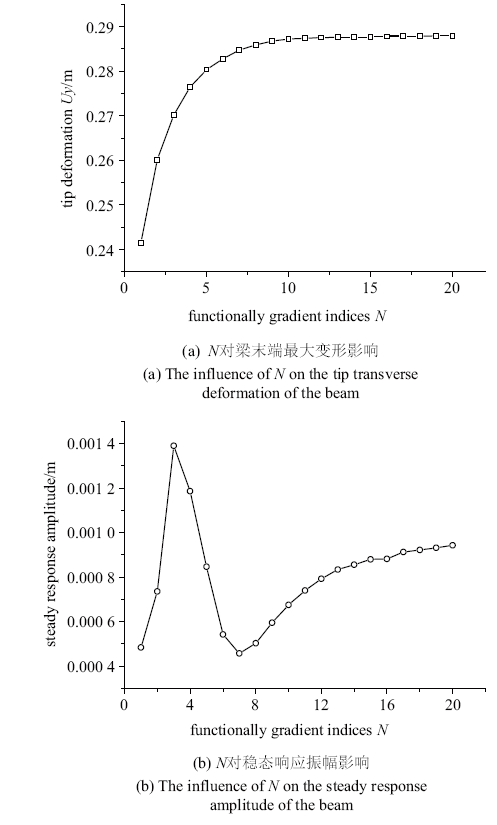Fig.7   Tip deformation and steady response amplitude of the beam under the influence of the different $N$

### 2.2 大范围运动未知情况下的动力学仿真

$\begin{eqnarray} \tau (t) = \left\{\begin{array}{ll} \tau _0 \sin \left( {\dfrac{2{\pi }}{T_{\rm t} }t} \right), \ \ & 0 \leqslant t \leqslant T_{\rm t} \\ 0, & t > T_{\rm t} \\ \end{array} \right. \end{eqnarray}$

### 图8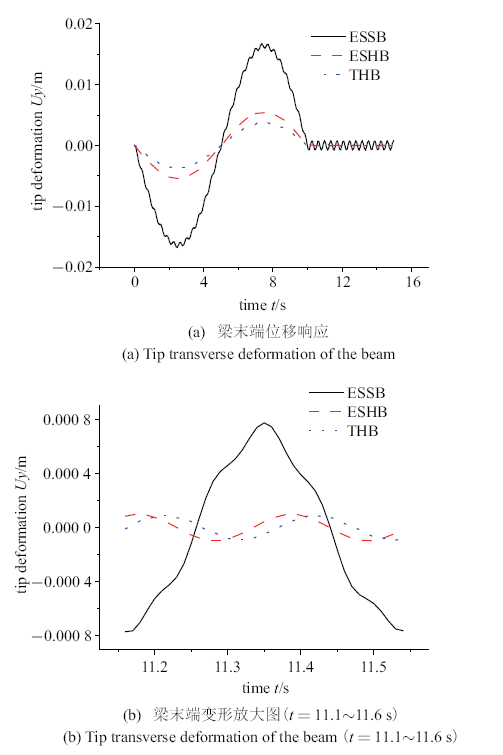Fig.8   Comparison of tip deformation of flexible beam in three different models

### 图9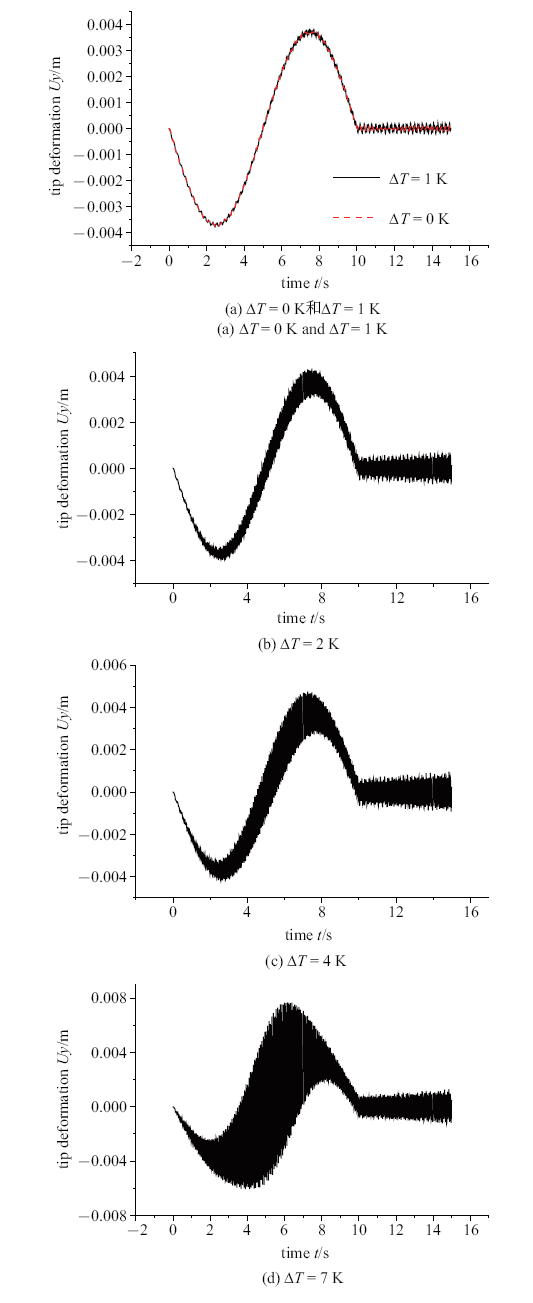Fig.9   Tip deformation of flexible beam under the influence of the different thermal shock load

### 图10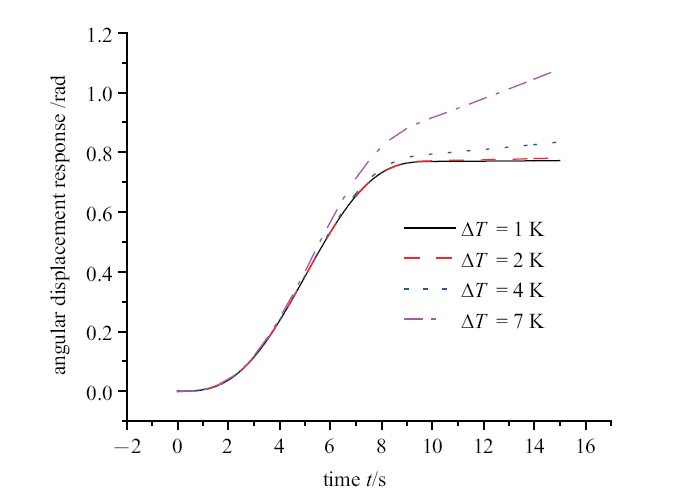Fig.10   Angular displacement response of the hub under the influence of the temperature

### 图11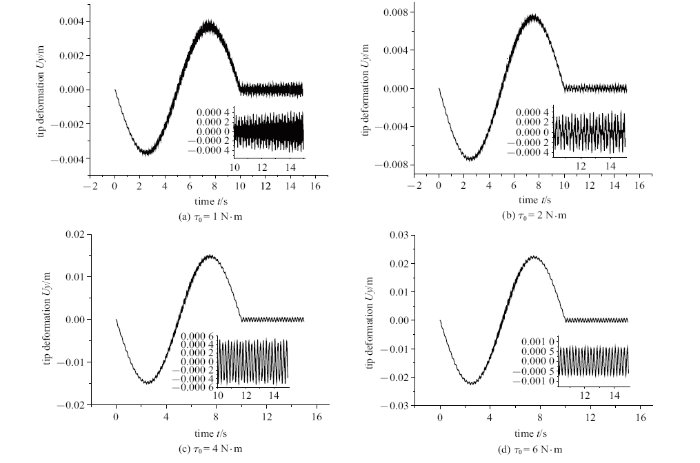Fig.11   Tip deformation of flexible beam under the influence of the different $\tau _{\rm 0}$

### 图12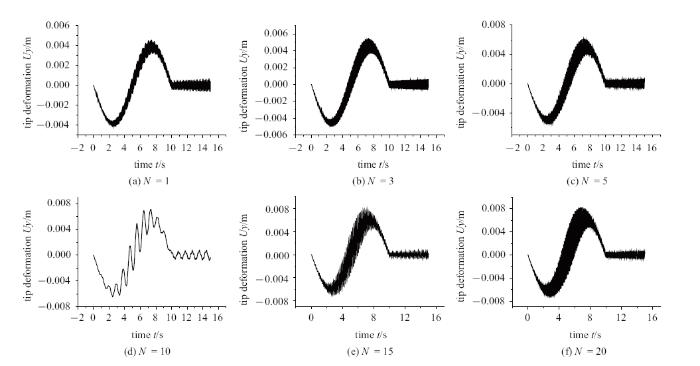Fig.12   Tip deformation of flexible beam under the influence of the different $N$

## 3 结论

(1)不同的结构形式对于柔性梁的动力学响应有较大影响,通过对比发现等截面实心梁和变截面空心梁两种简化模型差异较大,在进行分析计算时不能简单的等效.

(2)大范围运动已知时柔性梁在热冲击载荷作用下, 由于高温处陶瓷热膨胀系数小,低温处金属热膨胀系数大, 同时功能梯度梁的材料为对称分布形式,梁的横向弯曲变形被抑制, 且抑制作用随温度增大而增强;而大范围运动恒定时随着柔性梁内外温差增大, 热冲击的作用进一步叠加,进而产生高频振荡.

(3)当柔性梁由外力矩驱动和热冲击载荷作用时, 大范围运动未知是由较小的外力矩驱动,此时热冲击和外力矩产生热力耦合效应, 由于热力耦合的作用,在运动的初期就呈现高频振荡, 即柔性梁弹性变形和热冲击载荷产生耦合效应;随着外力矩的撤销, 柔性梁在热冲击载荷作用下继续高频振荡, 且振幅增大,随之带动中心刚体继续转动,即说明柔性梁弹性变形、热冲击载荷产生的热效应和中心刚体大范围旋转运动产生了刚柔热耦合作用.

(4)功能梯度指数$N$对两种运动的影响效果也不尽相同, 对于大范围运动已知主要在$N$较小时对其变形的大小产生作用, $N$过大对变形的影响已经不再明显; 对于大范围运动未知时, 合适的$N$将有效减小外部激励对柔性梁振荡的影响.

## 参考文献 原文顺序 文献年度倒序 文中引用次数倒序 被引期刊影响因子

Koizumi M.

FGM activities in Japan

Composites Part B: Engineering, 1997,28(1-2):1-4

( Jia Guangseng, Zhang Li, Lu Congming, et al.

Film cooling performance with internal coolant channel crossflow

Journal of Aerospace Power, 2015,30(4):823-830 (in Chinese))

( Li Guangchao, Mo Weishu, Zhang Wei, et al.

Experimental investigation on integrated colling performance of nozzle guide vane

Journal of Propulsion Technology, 2018,39(12):2772-2778 (in Chinese))

( Shi Xiaojun, Shui Linqi, Gao Jianmin, et al.

Internal cooling passage of mist/steam two-phase flow enhanced cooling gas turbine blade

Journal of Aerospace Power, 2015,30(11):2561-2567 (in Chinese))

Chowdhury NHK, Qureshi SA, Zhang M, et al.

Influence of turbine blade leading edge shape on film cooling with cylindrical holes

International Journal of Heat and Mass Transfer, 2017,115:895-908

Fan X, Li L, Zou J, et al.

Local heat transfer of vortex cooling with multiple tangential nozzles in a gas turbine blade leading edge cooling passage

International Journal of Heat and Mass Transfer, 2018,126:377-389

( Li Yanlin, Rao Yu, Wang Deqiang,

Numerical study on turbulent heat transfer of miniature ribs in turbine blade internal passage

Journal of Aerospace Power, 2018,39(10):161-169 (in Chinese))

( Qiu Qinggang, Shen Shengqiang,

Effect of Helix-Alike fin surface shape on flow and heat transfer in the internal cooling passage

Journal of Aerospace Power, 2006,21(1):71-76 (in Chinese))

( Qiu Qinggang, Wang Haipeng, Zhao Liang, et al.

Heat transfer and friction behavior in internal cooling duct with cuniform-fins

Journal of Aerospace Power, 2011,26(12):2648-2654 (in Chinese))

( Zhu Xingdan, Zhang JinZhou, Tan Xiaoming.

Effects of rotation on shaped-hole film cooling on a turbine blade

Journal of Propulsion Technology, 2016,37(9):1713-1719 (in Chinese))

( Zhu Xingdan, Zhang Jinzhou, Tan Xiaoming.

Composite cooling structure on rotating turbine blade

Journal of Aerospace Power, 2017(3):584-591 (in Chinese))

( Han Feng, Li Haiwang, Ma Yiwen, et al.

Effect of rotation on the leading-sdge region film cooling of a twisted turbine blade

Journal of Aerospace Power, 2019,34(6):1352-1363 (in Chinese))

Kane TR, Ryan R, Banerjee AK.

Dynamics of a cantilever beam attached to a moving base

Journal of Guidance, Control, and Dynamics, 1987,10(2):139-151

( Li Liang, Zhang Dingguo, Hong Jiazhen,

Dynamics of hub-functionally graded material beam systems

Journal of Mechanical Engineering, 2013,49(13):77-84 (in Chinese))

( Li Liang, Zhang Dingguo, Hong Jiazhen,

Dynamics of rectangular functionally graded thin plates undergoing overall motion

Journal of Dynamics and Control, 2013,11(4):329-335 (in Chinese))

( Fang Jianshi, Zhang Dingguo,

Rigid-flexible couping and frequency analysis of a rotating cantilever beam

Chinese Journal of Computational Mechanics, 2012,29(3):333-339 (in Chinese))

( Du Chaofan, Zhang Dingguo,

A meshfree method based on point interpolation for dynamic analysis of rotating cantilever beams.

Acta Phys. Sin, 2015,64(3):396-405 (in Chinese))

( Fan Jihua, Zhang Dingguo, Hong Jiazhen,

Dynamic analysis of a rotating tapered cantilever beam based on Bezier curve interpolation

Journal of Dynamics and Control, 2012,10(4):347-354 (in Chinese))

URL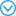( Fan Jihua, Zhang Dingguo,

Dynamic modeling and simulation of flexible robots based on different discretization methods

Chinese Journal of Theoretical and Applied Mechanics, 2016,48(4):843-856 (in Chinese))

URLURL( Fan Jihua, Zhang Dingguo.

Bezier interpolation method for the dynamics of rotating flexible cantilever beam

Acta Phys. Sin., 2014,63(15):154501-154501 (in Chinese))

URL( Fan Jihua, Zhang Dingguo,

B-spline interpolation method for the dynamics of rotating cantilever beam

Journal of Mechanical Engineering, 2012,48(23):59-64 (in Chinese))

( Han Guangcai, Wu Yanhong, Wang Yanchao, et al.

Dynamic analysis of a rotating blade with a rigid-flexible coupling, pre-twisted angle, and variable cross-section

Journal of Harbin Engineering University, 2011(6):736-741 (in Chinese))

URL( Chen Sijia, Zhang Dingguo,

Dynamics od hub-varable section beam systems

Chinese Journal of Theoretical and Applied Mechanics, 2011,43(4):790-794 (in Chinese))

URL( Gao Chentong, Li Liang, Zhang Dingguo, et al.

Dynamci modeling and simulation of rotating FGM tapered beams with shear effect

Chinese Journal of Theoretical and Applied Mechanics, 2018,50(3):210-222 (in Chinese))

Oh SY, Librescu L, Song O.

Vibration of turbomachinery rotating blades made-up of functionally graded materials and operating in a high temperature field

Acta Mechanica, 2003,166(1-4):69-87

( Wan Yunbo, Ma Rong, Wang Nianhua, et al.

Accurate aero-heating predictions based on multi-dimensional gradient reconstruction on hybrid unstructured grids

Chinese Journal of Theoretical and Applied Mechanics, 2018,50(5):1003-1012 (in Chinese))

( Xu Xin, Li Shirong,

Analysis of thermoelastic damping for functionally graded material micro-beam

Chinese Journal of Theoretical and Applied Mechanics, 2017,49(2):308-316 (in Chinese))

( Cao Leilei, Pei Jianzhong, Zhang Xuemin, et al.

Computer Simulation, 2012,29(8):387-390 (in Chinese))

( Liu Jinyang, Yuan Rui, Hong Jiazhen,

The on ridig-flexible coupling dynamicperformance for a rectangular plate considering thermal effect

Journal of Shanghai Jiaotong University, 2008,42(8):387-390 (in Chinese))

Cui YQ, Yu ZQ, Lan P.

A novel method of thermo-mechanical coupled analysis based on the unified description

Mechanism and Machine Theory, 2019,134:376-392

Cepon G, Starc B, Zupancic B, et al.

Coupled thermo-structural analysis of a bimetallic strip using the absolute nodal coordinate formulation

Multibody System Dynamics, 2017,41(4):391-402

URL( Li Shirong, Su Houde, Cheng Changjun,

Free vibration of functionally Graded material beams with surface-bonded piezeoelectric layers in thermal environment

Applied Mathematics and Mechanics, 2009,30(8):907-918 (in Chinese))

URL( Li Liang, Zhang Dingguo, Guo Yongbin,

Dynamics of rigid--flexible coupling Fgm beam systems in variable temperature fields

Journal of Vibration Engineering, 2017,30(1):9-19 (in Chinese))

Javaheri R, Eslami MR.

Thermal buckling of functionally graded plates based on higher order theory

Journal of Thermal Stresses, 2002,25(7):603-625

Chen Y, Jin G, Zhang C, et al.

Thermal vibration of FGM beams with general boundary conditions using a higher-order shear deformation theory

Composites Part B: Engineering, 2018,153:376-386

/

 〈〉